Problem C1.3. Flow over a NACA0012 Airfoil

Overview

This problem is aimed at testing high-order methods for the computation of external flow with a high-order curved boundary representation. Both inviscid and viscous, subsonic and transonic flow conditions will be simulated. The transonic problem will also test various methods’ shock capturing ability. The lift and drag coefficients will be computed, and compared with those obtained with lower order methods.

Governing Equations

The governing equation is the 2D Euler and Navier-Stokes equations with a constant ratio of specific heats of 1.4 and Prandtl number of 0.72. For the viscous flow problem, the viscosity is assumed a constant.

Flow Conditions

Three different flow conditions are considered:

a)     Subsonic inviscid flow with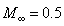, and angle of attack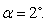b)     Inviscid transonic flow with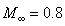, and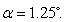c)     Subsonic viscous flow with, and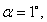Reynolds number (based on the chord length) Re = 5,000.

Geometry

The NACA0012 airfoil is defined in the following equation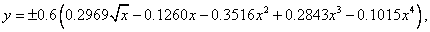where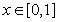. The airfoil defined using this equation has a finite trailing edge of .252%. Various ways exist in the literature to modify this definition such that the trailing edge has a zero thickness. We adopt the one which modifies thecoefficient, i.e.,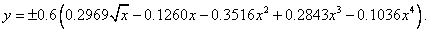The airfoil is shown in the following figure.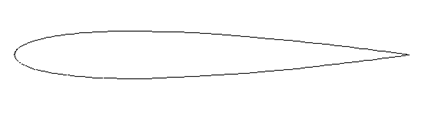Figure 1.3. NACA0012 Airfoil

Boundary Conditions

Far field boundary: subsonic inflow and outflow

Airfoil surface: slip wall for inviscid flow, or no slip adiabatic wall for viscous flow

Requirements

1.     If you generate new meshes, please adhere to the following guideline: The far field should be a circle, centered at the airfoil mid chord with a radius of 1000 chords. Do not apply any vortex correction at the far field.

2.     Start the simulation from a uniform free stream everywhere, and monitor the L2 norm of the density residual. Compute the work units needed to achieve a steady state. Compute the lift and drag coefficients cl and cd.

3.     Perform hp-refinement studies to find “converged” cl and cd values with an error of 0.01 count.

1.     Plot the cl and cd errors vs. work units for different h and p.

2.     Study the numerical order of accuracy according to cl and cd errors vs.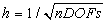3.     Submit two sets of data to the workshop contact for this case

a.      cl and cd errors vs. work units for different h and p

b.     cl and cd errors vsfor different h and p